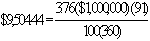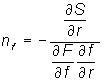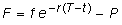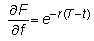﻿ 6.7 Hedging with Futuresoffice (412) 9679367
fax (412) 967-5958
toll-free 1 (800) 214-3480

6.7 Hedging with Futures

 I

n our exposition of duration, the exposure of a position to interest rate risk is managed by controlling the duration of the hedged position. In fact, a duration-zero position is insensitive to parallel shifts in the yield curve. We have assumed the duration of the position is managed using bonds, but duration also can be managed using futures.

Recall from Chapter 2 that the arbitrage-free delivery price of a future equals the future value of the underlying spot price. For example, if the spot price is P and the futures contract is settled in two periods’ time, then the futures price is:Assuming compounding in continuous time, the futures price is again the future value of the underlying spot at the time of delivery:where r is the instantaneous yield to maturity that applies over the life of the futures contract.

An important distinction is made between the futures price, f, which is the delivery or settlement price, and the present value of a futures contract, F, which is an obligation to exchange f for the underlying security at the time of settlement. The present value of the futures contract is zero when the futures price is arbitrage-free, because no cash is exchanged at the time of buying or selling the futures contract. You should note, however, that subsequent to fixing f, the present value of the futures contract, F, will fluctuate with changes in interest rates.

Now suppose we are using futures contracts to hedge some underlying position that has a spot value equal to S. The objective is to use the valuation model to construct the appropriate hedge ratio for futures contracts.

Let the hedged position be expressed as:where F is the value of the futures contract. Now each of these values is a function of the current instantaneous yield to maturity, r, so we are interested in the change in the value of this hedge as a function of r. Using the chain rule we get:The appropriate hedge ratio nf is such that the left hand side's partial derivative equals zero. That is, the value of the hedge is insensitive to small changes in the yield to maturity. This implies thatNow F is the present value of the futures contract:Simplifying this equalsThus,Recall that, initially, the arbitrage-free present value of the futures price is zero, but as subsequent shifts occur in interest rates, this will no longer be the case. If the shifts in interest rates are shifts in forward rates subsequent to the time of settlement of the futures contract, then this assumption is reasonable.In the next topic, Application to a Futures Example, we will apply this theory to numerically construct a futures hedge.